# Types of Determinants and Their Properties JEE Notes | EduRev

## JEE : Types of Determinants and Their Properties JEE Notes | EduRev

The document Types of Determinants and Their Properties JEE Notes | EduRev is a part of the JEE Course Mathematics (Maths) Class 12.
All you need of JEE at this link: JEE

F. DETERMINANT

(i) Submatrix : Let A be a given matrix. The matrix obtained by deleting some rows or columns of A is called as submatrix of A.

e.g.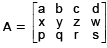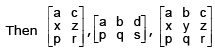are all submatrices of A.

(ii) Determinant of A Square Matrix :

Let A [a]1 x 1 be a 1 x 1 matrix. Determinant A is defined as |A| = a  eg. A = [–3]1 x 1  |A| = –3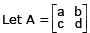,then |A| is defined as ad –bc.

(iii)Minors & Cofactors : Let Δ be a determinant. Then minor of element aij, denoted by Mij is defined as the determinant of the submatrix obtained by deleting ith row & jth column of Δ.

Cofactor of element aij, denoted by Cij is defined as Cij = (–1)i + j Mij.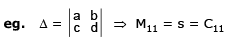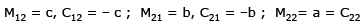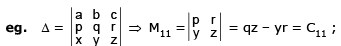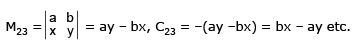(iv)Determinant : Let A = [aij]n be a square matrix (n > 1). Determinant of A is defined as the sum of products of elements of any one row (or one column) with corresponding cofactors.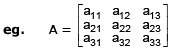|A| = a11C11 + a12C12 + a13C13 (using first row)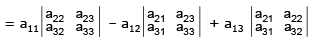|A| = a12 C12 + a22C22 + a32C32 (using second column)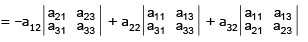G. PROPERTIES OF DETERMINANTS

P- 1 : The value of a determinant remains unaltered , if the rows & columns are inter changed . e.g. If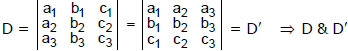are transpose of each other.

If D' = - D then it is SKEW SYMMETRIC determinant but D' = D ⇒ 2 D = 0 ⇒ D = 0 ⇒ Skew symmetric determinant of third order has the value zero .

P-2 : If any two rows (or columns) of a determinant be interchanged , the value of determinant is changed in sign only . e.g.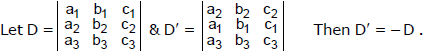P-3 : If a determinant has any two rows (or columns) identical , then its value is zero.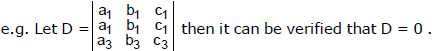P-4 : If all the elements of any row (or column) be multiplied by the same number then the determinant is multiplied by that number.

e.g. If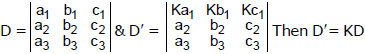P-5 : If each element of any row (or column) can be expressed as a sum of two terms then the determinant can be expressed as the sum of two determinants.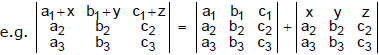P-6 : The value of a determinant is not altered by adding to the elements of any row (or column) the same multiples of the corresponding elements of any other row (or column).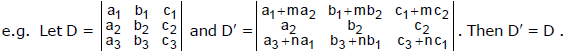Note that while applying this property atleast one row (or column) must remain unchanged.

P- 7 : If by putting x = a the value of a determinant vanishes then (x-a) is a factor of the determinant

Ex.17 Find the value of the determinant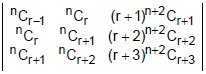Sol.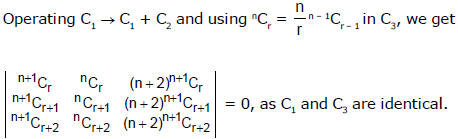Ex.18  A is a n × n matrix (n > 2) [aij] where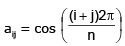Find determinant A.

Sol.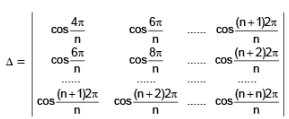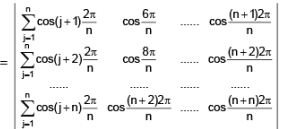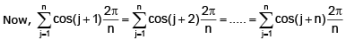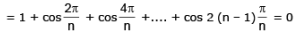⇒ value of determinant is zero.

H. MULTIPLICATION OF TWO DETERMINANTS

(i)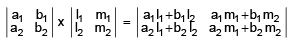Similarly two determinants of order three are multiplied.

(ii)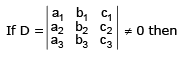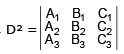where Ai , Bi , Ci are cofactors

PROOF : Consider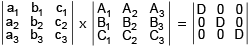Note : a1A2 + b1B2 + c1C= 0 etc.

therefore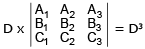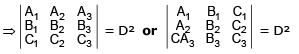Ex.19 Prove that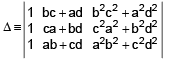= (a – b) (a – c) (a – d) (b – c) (b – d) (c – d).

Sol.

Applying R2 → R2- R1, R3 → R- R1, we get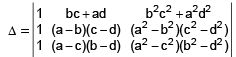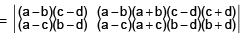=(a – b) (c – d) (a – c) (b – d)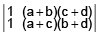= (a – b) (c – d) (a – c) (b – d) [(a + c) (b + d) – (a + b) (c + d)]

= (a – b) (c – d) (a – c) (b – d) (ab +cd – ac – bd) = (a – b) (a – c) (a – d) (b – c) (b – d) (c – d).

Alternatively :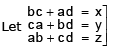and using c3 → c3 + 2 a b c d . c3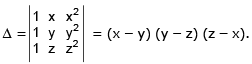Ex.20 Show that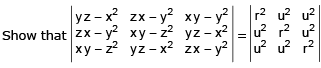(where r2 = x2 + y+ z2 & u2 = x y + y z + z x)

Sol. Consider the determinant ,We see that the L.H.S. determinant has its constituents
which are the co-factor of Δ. Hence L.H.S. determinant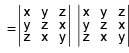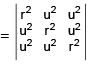Ex.21 Without expanding, as for as possible, prove that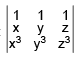= (x – y) (y – z) (z – x) (x + y + z)

Sol.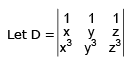for x = y, D = 0 (since C1 and C2 are identical)

Hence (x – y) is a factor of D (y – z) and (z – x) are factors of D. But D is a homogeneous expression of the 4th degree is x, y, z.
∴ There must be one more factor of the 1st degree in x, y, z say k (x + y + z) where k is a constant.
Let D = k (x – y) (y – z) (z – x) (x + y + z),Putting x = 0, y = 1, z = 2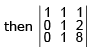= k (0 – 1) (1 – 2) (2 – 0) (0 + 1 + 2)

⇒ L(8 – 2) = k(–1) (–1) (2) (3) ∴ k = 1 ∴ D = (x - y) (y - z) (z - x) (x + y + z)

Ex.22 Prove that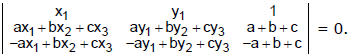Sol.

Given that Δ =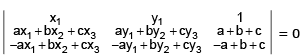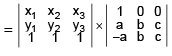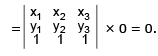Ex.23 Express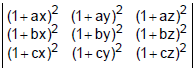as product of two determinants.

Sol. The given determinant is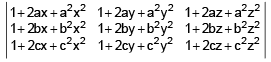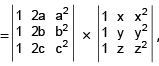with the help of row–by–row multiplication rule.

Ex.24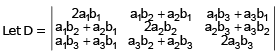Express the determinant D as a product of two determinants. Hence or otherwise show that D = 0.

Sol.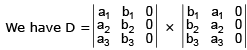as can be seen by applying row–by–row multiplication rule., Hence D = 0.

Offer running on EduRev: Apply code STAYHOME200 to get INR 200 off on our premium plan EduRev Infinity!

## Mathematics (Maths) Class 12

209 videos|222 docs|124 tests

,

,

,

,

,

,

,

,

,

,

,

,

,

,

,

,

,

,

,

,

,

;